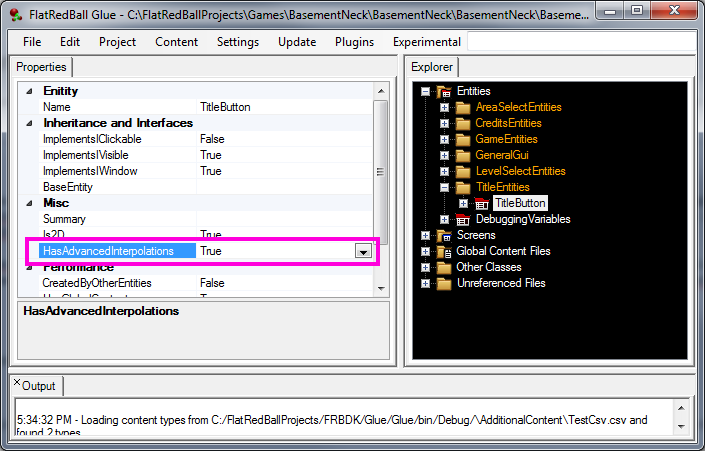## Introduction

The Advanced Interpolation Plugin simplifies advanced interpolation between States. This plugin provides numerous interpolation methods which are applied using the standard InterpolateBetween method provided by Glue. The logic generated by this plugin is available through an overload to the InterpolateToState method.

Just like the regular InterpolateToState method, the method generated by this plugin needs to only be called one time and interpolation will automatically happen over time with no additional custom code needed. In other words, if you are familiar with the InterpolateToState method provided by Glue, you will find this method very similar in usage.

## What is State Interpolation?

If you have been using Glue for your projects then you may be familiar with states. If not, you should start by checking out the States page.

Since states can set numerical values, that means that multiple states can be combined to create an intermediary state at runtime. For example, consider a State called “Transparent” which sets the Alpha on a Sprite to 0. Also, consider a second State called “Opaque” which sets the Alpha on a Sprite to 1. These two states can be combed to create values inbetween 0 and 1 for the Sprite’s Alpha. This is the basics behind State Interpolation. For a more complete discussion, see this page and this page.

## What Are the Different Kinds of Interpolations

A visualization of the different interpolations can be found on this page.

## Creating Interpolation Endpoints

Interpolation endpoints are the “start” and “end” of your interpolation. These endpoints are defined using states. For example, you may expose the X value on an Entity, create two states – each of which sets a different X value. Once this is done, you can interpolate between the two states. Common values used in interpolation are:

• Position values such as X, Y, and Z
• Alpha values for fading out/in
• Rotation values
• Scale values